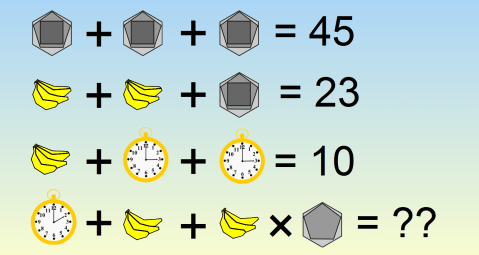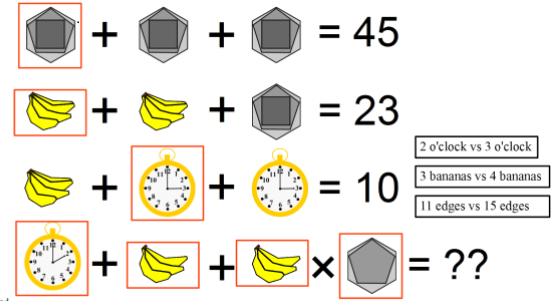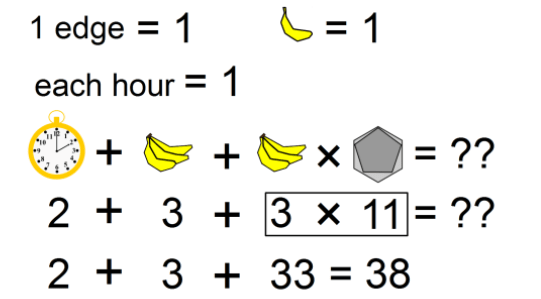# Hexagon Banana Clock Riddle

This riddle is making people go crazy! One of the most viral riddles of all time. We bet even your class’ smartest person will have trouble solving this but here we are giving you the answer with the explanation so that you can brag and be the real Einstein of your class 🙂Here is the riddle:

Hexagon + Hexagon + Hexagon = 45
Bananas + Bananas + Hexagon = 23
Bananas + Clock + Clock = 10
Then,
Clock + Bananas + Bananas x Hexagon = ??

Think Logically!

Hints :

Look closely at the clock digits/ the number of Bananas/ sides of the hexagons.

Answer is not 19 or 26 🙂

## Hexagon Banana Clock Riddle Answer

First spot the differences in last line.Let’s solve value of each shape:

Hexagon + Hexagon + Hexagon(3 Hexagon) = 45

1 Hexagon = 15 (Remember that total edges in this hexagon, pentagon and square are 6 + 5 + 4 = 15)

Next is

Bananas + Bananas + Hexagon = 23

Bananas + Bananas = 23 – Hexagon = 23 – 15 = 8

Bananas = 8/2 = 4

Each Banana pic has 4 bananas so each Banana value is equal to 1

Next is

Bananas + Clock + Clock = 10

4 + 2 (Clock) = 10

Clock = 6/2 =3

Notice the clock hours(3 o’clock)  which is means each hour is equal to 1

Now last equation

Clock + Bananas + Bananas x Hexagon = ??

There are 4 things to notice:

1. Clock time is 2 o’clock not 3 o’clock
2. There are only 3 bananas compared to 4 in other equations
3. Hexagon has missing square shape
4. There is multiplication between Bananas and Hexagon2(2 o’clock) + 3 (3 bananas) + 3 (3 bananas) X 11 (11 edges)

2 + 3 + 33 = 38

Also Try: Spot the differences puzzle

Search Related Keywords:

Banana Riddle

Clock Banana Hexagon Riddle

Hexagon Banana clock Riddle